• 검색 결과가 없습니다.

# Area and Power Efficient 10-Bit Column Driver with 67-MS/s Time-Dividing DAC and Output Buffer

N/A
N/A
Protected

Share "Area and Power Efficient 10-Bit Column Driver with 67-MS/s Time-Dividing DAC and Output Buffer "

Copied!
59
0
0
더 보기 ( 페이지)

전체 글

Structure of a DAC with a series of resistors and resistors without intermediate buffers Image of a DAC with a series of resistors and resistors. a) Offset error and (b) DAC gain error. Monotonic characteristic of DAC and (b) non-monotonic characteristic of DAC Figure INL DAC with respect to input digital data. Relationship between non-linear gamma correction and interpolation technique Fig. A common column driver IC structure

Charge injection error induced when sampling switch is turned off Fig. DEMUX with sample and hold circuit. Input stage of Class AB amplifier with source follower. a) Output stage of the output buffer amplifier (b) Voltage gain specification. a) Data management timing diagram of conventional and proposed display column driver IC Fig. a) Monotonicity simulation of proposed high-speed DAC.

## Conventional Display Column Driver IC

As shown in figure 3, the conventional column driver IC is divided into digital blocks consisting of shift registers, sampling latches and hold latches, and analog blocks consisting of level shifter, DAC and output buffer , . Because the digital parts use low voltage elements for 1.8V, the data stored in the hold latch has a value of 0V or 1.8V. In order to meet the voltage range used for display panel operation, the low voltage of the digital parts is converted to a high voltage value of 0V or 5V.

## DAC basics and architectures

Relationship between resolution of DAC and image quality

### DAC architectures

• Resistor-string DAC(R-DAC )
• Cyclic DAC
• Current-steering DAC
• Resistor-string with Embedded DAC
• Resistor-Resistor string DAC(RR-DAC)
• Resistor-Resistor string DAC without intermediate buffers
• Resistor-Floating-Resistor string DAC

Resistor string with embedded DAC consists of a total of two stages using interpolation scheme, so the first stage is an R-DAC consisting of a resistor string, and the second stage is an output buffer with embedded DAC, so the data conversion procedure can be performed only by changing a part of the output buffer without additional DAC structures -. Figure 10 shows a 10bit resolution DAC with a 7bit resistor string DAC on the first stage and a 3bit embedded DAC on the second stage. First, two adjacent voltages VH, VL are generated in accordance with the upper 7-bit digital data input to the first 7-bit resistor string DAC.

As with the above resistor string with built-in DAC, the difference between the two adjacent voltages, VH and VL that is from the first stage, is divided into a certain number of levels according to the resolution set in the second stage by the interpolation scheme. Although the RR-DAC has the advantage of being divided into two stages and can be applied in a small area, the problem is that the loading effect occurs in the first stage from the second stage of the resistor string. However, the loading effect is still caused by the impedance string of each channel, which also has problems with accurate data conversion.

In this structure, the current flows in each channel resistance string through the compensation current source and current -. This current is the same as the current flowing through each channel from the two adjacent voltages VH and VL from the first stage of the global resistance string. At the top of the channel resistance range, the current source drains the current into each channel as a value of e.

The channel resistor array is called Floating-Resistor DAC because the same current flows in and out, and it is the most efficient structure in terms of area efficiency and uniformity between the channels among the various DAC forms mentioned so far.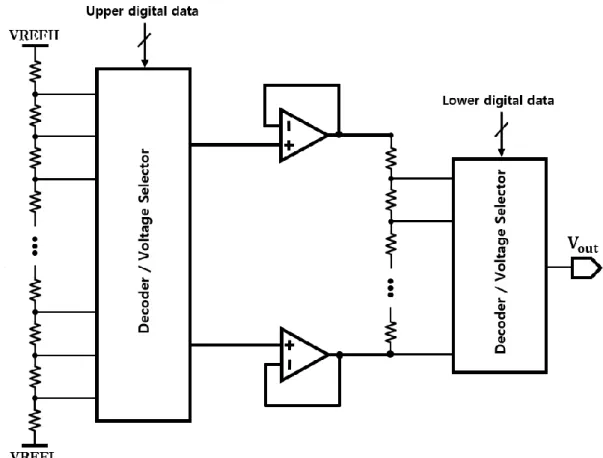Figure 11 shows the structure of the Resistor-Resistor string DAC, with both the first and second sections in the form of the resistor-string

### DAC Specification

• Offset error and Gain error
• Monotonicity
• Integral Non-Linearity(INL) and Differential Non-Linearity(DNL)

INL is a difference between the analog output voltage value of the ideal DAC and the analog output voltage value of the actual DAC compared to a Least Significant Bit (LSB) on the same digital data input. And DNL is an index that represents the difference between the step size of the ideal DAC and the actual DAC as depicted in figure 17. As such above, INL and DNL are indicators of how the output values ​​of the actual DAC differ from the output values of the ideal DAC, so they are one of the important figures to represent the performance of the DAC.

The path from each current source to the power VDD has a wire resistance of 𝑅, which means that the transistors are looking at different values ​​of resistance in the direction of VDD, so they have different voltage drops. Consequently, although both the dimension and bias of the transistors are the same, there is a slight difference in the actual current flow and the error that occurs in the structure of these systems is called systematic error. Finally, a design technique is used to reduce the influence of random error and gradient error to improve the static performance in the case of a resistor array DAC .

First, simply the dimensions of the resistors can be large to reduce random errors, but this is not efficient in terms of increasing the area of ​​the whole chip, since many resistors are used in the resistor string DAC. In other ways, N extra bits of accuracy of M = 4𝑁 can be obtained by connecting M sub-resistors of the same dimensions as in figure 20-(a) in parallel. Assuming that each unit resistance is R, the effective resistance can be expressed as R = R0(1+∈) where R0 is the value of the ideal unit resistance and ∈ is the value that reflects the random error occurring during the manufacturing process.

As an improvement method, the four-unit resistors can be connected in series and parallel as shown in Figure 20-(b) to achieve the effect of an extra bit of accuracy above without changing the effective actual resistance value.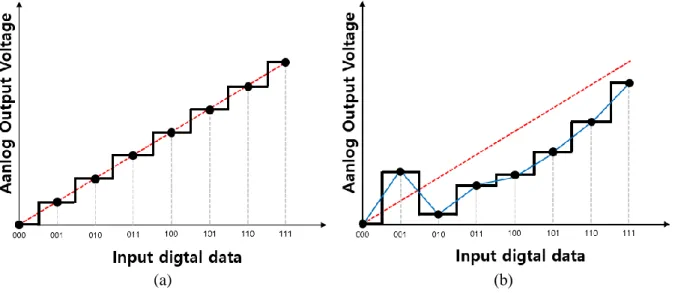Figure 15 shows the case where DAC is monotonic or non-monotonic. The DAC's characteristic of monotonicity means that as input digital data values increase, the output analog voltage also increases, and vice versa, the output analog voltage decreases as

## Structure and Operation of Proposed Column Driver IC

In this thesis, the interpolation was done in two phases, taking into account the short 1-H time of the 5.48us. In addition, 6bit digital data for the first stage and 4bit for the second stage were allocated for implementation with a smaller area, and the linear gamma correction method was applied. The conventional R-DAC based column driver IC uses one R-DAC and one output buffer amplifier for each column channel.

This is to match the data conversion and the final 1-H time limited analog signal due to the settling time of the DAC and the normal speed output buffer amplifier. Analog signals from one R-DAC are applied to one output buffer amplifier and the final output voltage is applied to each column channel and plate load as shown. In the proposed column driver IC, 24 column channels are shared by one high-speed DAC, and two column channels are shared by one output buffer amplifier, as shown in Figure 25.

More specifically, among the converted 24 analog data, the first 12 data, data1~data12, are stored in 12 sample capacitors, cap1~cap12, and once they are stored, sample capacitors are connected to 12 output buffer amplifiers, amp1~ amp12 respectively to load1~load12 drive sequentially. After load shedding in the 12 channels is completed, the remaining 12 data, data13~data24, are stored in sample capacitor, cap1~cap12 again, and like the previous process, each sample capacitor is connected to amp1~amp12 and load13~load24 is driven sequentially.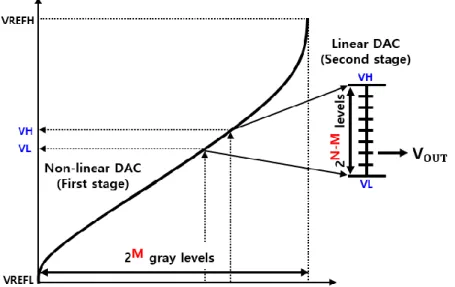Figure 24 shows the structure of the conventional column-driver IC with R-DAC of a typical data conversion speed

## Circuit Implementation

D flip flop

### High speed DAC

For fast data conversion, the ROM type structure is adopted in the 6bit voltage selector of the first stage. The second stage of the 4bit voltage selector is also implemented in ROM type with a small RC delay. In the output buffer array, output voltages from the DAC sampled on the capacitor of the previous stage are applied sequentially to the panel load via the output buffer amplifier.

For the application of the output buffer dividing technique, two output switches are connected to each buffer amplifier as shown in figure 37. When designing the output buffer amplifier, it is important to note that the slow settling speed of the negative terminal affects the fast settling speed of the positive terminal through the parasitic capacitor of the input terminal as shown in figure 38. And the settling time of the output buffer amplifier is 1.8us when 6.5kohms and 35pF panel load is connected.

The phase edge has a result of 56 degrees and has the characteristic of stable voltage regulation. a) Output stage of the output buffer amplifier (b) Voltage gain specification. As shown in Figure 43-(a), in the case of the normal type, all channels have their own DAC and output buffer, so the DAC and output buffer setpoints are the same on all channels. In contrast, in the case of the proposed type as in Figure 43-(b), 24 channels from channel 1 to channel 24 use the same DAC and 2 channels use the same output buffer amplifier.

The proposed 24-column time division DAC and output buffer part display driver IC for Active Matrix Organic Light Emitting Diode (AMOLED) column driver applications aims to improve the overall power and area efficiency of the IC.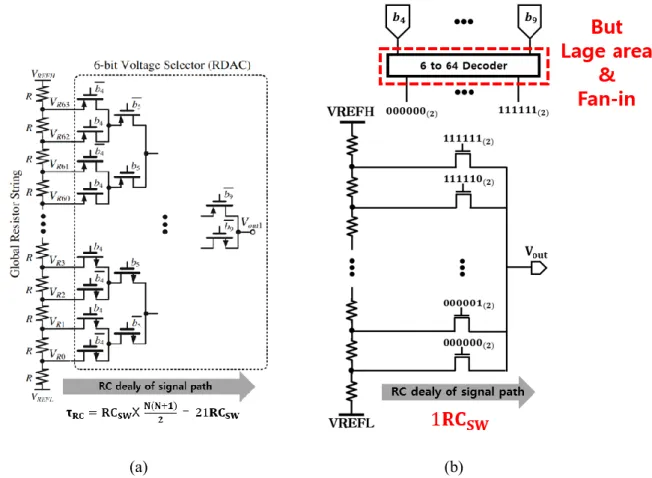Figure 30 shows a comparison of the RC delay in the signal path of the tree type and ROM type voltage selector

Output Buffer Array

## DAC settling time

The target 1-H time is 5.48 us, so the number of 24 is the maximum value for the division of a DAC.

Rail to Rail Class AB output buffer amplifier

Timing diagram of proposed display column driver IC

DAC’s monotonicity characteristic

Full chip layout design

## Measurement floor plan

A new technique of dividing the DAC and output buffer amplifier by dividing the limited 1-H time into the timing allows more efficient use of the 1-H time compared to traditional display column driver ICs. Lin, “A 10-bit Two-Stage R-DAC with Isolating Source Followers for TFT-LCD and AMOLED Column-Driver ICs,” IEEE Trans. Huang, “A 10-bit LCD column driver with piecewise linear digital-to-analog converters,” IEEE J,Solid-Stage Circuits, vol.

Chung, “A high-voltage, high-speed, low-power rail-to-rail driver for 8-bit large-scale TFT LCD applications,” IEEE Trans. Jeon et al., "Partially linear 10-bit DAC architecture with drain current modulation for compact LCD driver ICs," IEEE J. Choi, "Low-area 10b column driver with resistor-resistor-array DAC for mobile LCDs with active matrix ,” 2016 IEEE Asia-Pacific Conference on Circuits and Systems (APCCAS), p.

Chang, “A 10-bit Resistor Floating Resistor String DAC (RFR-DAC) for High Color Depth LCD Driver ICs,” IEEE J. Ismail, “Design Techniques for Improving the Intrinsic Accuracy of Resistor String DACs ", IEEE International Symposium on Circuits and Systems, 2001. H Cho, "A 10-bit column-driver IC with parasitic-insensitive iterative charge-sharing based capacitor-string interpolation for mobile active-martix LCDs", IEEE J.

Huijsing, “A compact power-efficient 3 V CMOS rail-to-rail I/O operational amplifier for VLSI cell libraries,” IEEE J .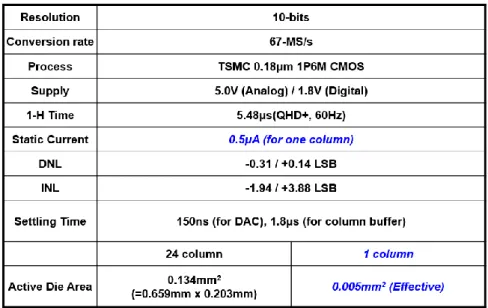Table 1 shows overall specification of proposed column driver IC. The number of columns sharing one DAC is 24, with a die size of 0.134mm 2

수치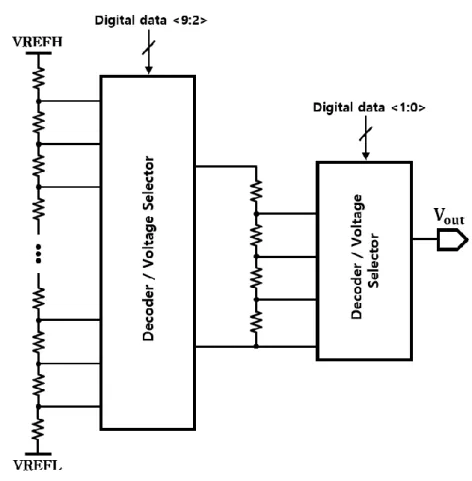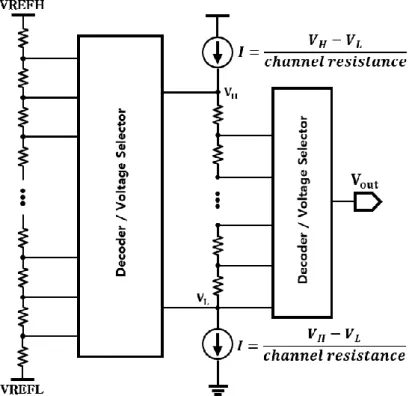+7

참조

관련 문서

The developed driver consists of two 5-bit resistor-string DACs, and an analog buffer which has an analog voltage adder function.. The area of the proposed DAC takes only

The proposed scheme achieves a high-speed and high-perfor- mance VLC by exploiting the diversity gain (for high perfor- mance) and color-clustered multiplexing (for high speed)

ALVV is the control that regulates link voltage variation proportional to the output voltage variation to maintain unity dc gain of the LLC

(Refer to pages 4 and 5 for details.) If your amplifier does not have any line output terminal, connect the subwoofer to the speaker output terminals of the amplifier.. (Refer

The column system designed by the proposed method reduced reboiler energy consumption and total annual cost by 28.23 % and 25.49 % , respectively, in case 1, and those by 25.63 %

In this paper, effect of power/ground bounce noises on the operational amplifier is analyzed and PDN models of the package, chip and circuit are proposed to analyze the DC

The proposed configurable digital controller provides high reliability and accuracy by detecting and ensuring the number of configurable continuous wake-up signal

Using a loop-gain stabilizer in LDO, the gain of the error amplifier can be constant in a wide range of load current or reference voltage.. The LDO achieves high PSR and fast

The proposed receiver consists of a low-noise transcondutance amplifier LNTA, single-balanced current-mode passive mixer, transimpedance amplifier TIA, variable gain amplifier VGA, and

언어 선택하기

웹사이트는 선택한 언어로 번역됩니다.

기타 언어